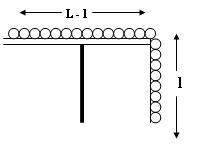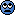# Lagrangian of System

## Homework Statement

Uniform chain of length L is kept of a horizontal table in such a way that l of its length keeps hanging from the table. If the whole system is in equilibrium, find the Lagrangian of the system.## Homework Equations

Lagrangian of the system = Kinetic energy (T) - Potential energy (V)
T = (mv^2)/2
V = mgh

## The Attempt at a Solution

In this case, V = 0 - mgl = -mgl
My question is what will be the kinetic energy of the system ?

I think as the system is in equilibrium, there is no motion in any portion of the chain. So, K.E. = 0
Am I wrong ?

---------------------------

What was that @Dilemma Can you please fill in the blanks ?I tried to solve your problem calculating the forces acting on the system but then I noticed that you were using the energy changes of the system. However, if I were you, I would introduce a friction force of μ to the system. There is no way this system can stay like that.

hilbert2